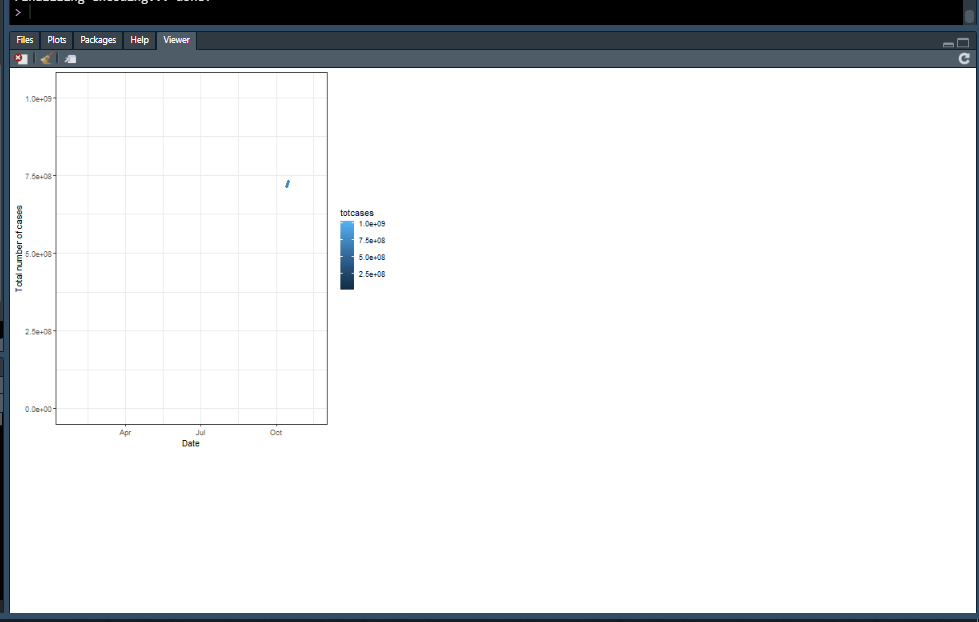# R viewer margins issue

My Rstudio viewer has something wrong with its margins. it produces small margins outputs. I tried

``````par(mfrow = c(1,1))
``````

but it didn't help. the problem is best explained by the following pictureThe code that I want to display on the viewer is

``````ggplot(us_cases, aes(x = myDate, y = totcases, color = totcases)) + geom_point() +
labs(x = "Date", y = "Total number of cases") +
theme_bw() + transition_time(myDate)
``````